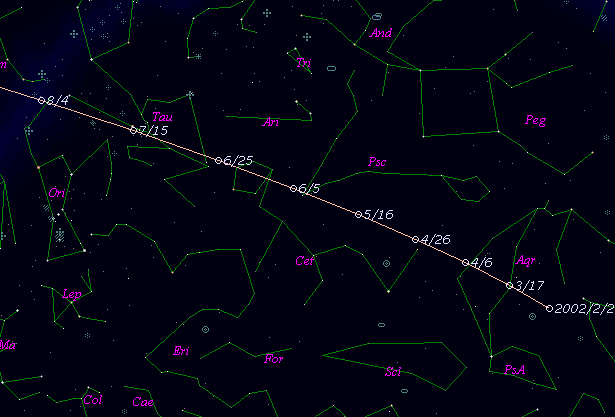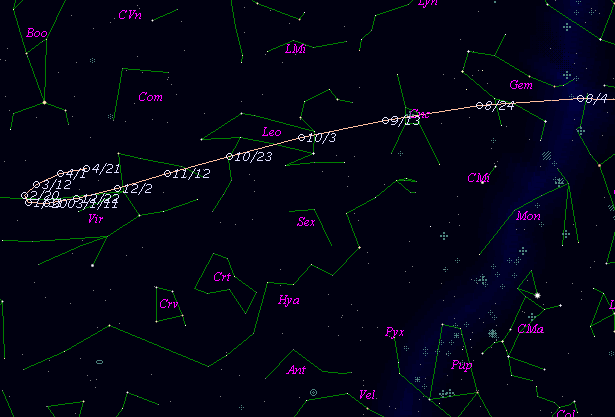# 46P/Wirtanen (2002)###Orbital Elements

```Epoch 2002 Sept. 3.0 TT = JDT 2452520.5
T 2002 Aug. 26.74628 TT                                 MPC
q   1.0587602            (2000.0)            P               Q
n   0.18107117     Peri.  356.39842     +0.19682227     -0.95950035
a   3.0943529      Node    82.17432     +0.90454239     +0.09840414
e   0.6578412      Incl.   11.73813     +0.37823810     +0.26396157
P   5.44
From 252 observations 1995 June 26-2002 June 23, mean residual 0".61.  Non-
gravitational parameters A1 = +0.23 +/- 0.03, A2 = -0.1892 +/- 0.0097.
```

###Finding Charts###Magnitudes Graph

```        m1 = 14.5 + 5 log d +  8.0 log r  [   ,-60]  (             - 2002 June 27)
m1 =  7.3 + 5 log d + 70   log r  [-60, 29]  (2002 June 27 - 2002 Sept.24)
m1 =  3.6 + 5 log d + 70   log r  [ 29, 55]  (2002 Sept.24 - 2002 Oct. 20)
m1 =  8.4 + 5 log d + 25.0 log r  [ 55,   ]  (2002 Oct. 20 -             )
```##### The orbital elements are calculated by Dr. Brian G. Marsden and printed on M.P.E.C. 2002-M33. The charts are made with StellaNavigator Ver.2.0 for Windows (AstroArts / ASCII). The magnitudes graphs are made with Comet for Windows.